# Short Answers - Gravitation, Science, Class 9 Notes | Study Class 9 Science by VP Classes - Class 9

## Class 9: Short Answers - Gravitation, Science, Class 9 Notes | Study Class 9 Science by VP Classes - Class 9

The document Short Answers - Gravitation, Science, Class 9 Notes | Study Class 9 Science by VP Classes - Class 9 is a part of the Class 9 Course Class 9 Science by VP Classes.
All you need of Class 9 at this link: Class 9

Q1. During free-fall what is the weight of a body. Give reason for the answer.
Ans.
During free-fall the body gets accelerated. This acceleration is caused due to the earth’s gravitational force. Hence the weight of the body is ‘0’.

Q2. Mass of a dog on earth is 40 kg, what is its mass on the moon?
Ans.
Mass on earth = 40 kg.
Mass of the body is its inertia, which remains constant.
∴ Mass of the dog on moon = 40 kg.

Q3. Obtain a relation between ‘g’ and ‘G’.
Ans.
Consider an object of mass ‘m’ placed at the surface of earth. Their force of gravitation acting on the object due to earth is given by, where M = mass of earth and
R = radius of earth By Newton’s second law
F = mg, where
g = acceleration of given object due to gravitational pull of earth.

mg = GMm/ R2

g = GM/R2

Q4. Distinguish between force of gravity and gravitational force.
Ans.
By gravitational force we mean force of gravitational attraction between any two objects in the universe whether the objects are light or heavy and irrespective of the distance between them.
Force of gravity is simply a special case of gravitational force and it means force of attraction between the object and the earth.

Q5. The mass of the body on earth is 60 kg what is its weight on earth and on moon?
Ans.
Mass of the body on earth = 60 kg Weight on earth = ?
W = m × g (g = 9.8 m/s2) = 60 × 9.8
= 588 N.
Weight on moon = (1/6)th the weight on earth
= (1/6) × 588
= 98 N.

Q6. The mass of earth is 6 × 1024 kg and that of moon is 7.4 × 1022 kg. If the distance between the earth and the moon is 3.84 × 10km. Calculate the force exerted by the earth on the moon. G = 6.67 × 10–11 Nm2 kg–2.
Ans.
It is given that the mass of earth Me = 6 × 1024 kg
Mass of moon Mm = 7.4 × 1022 kg
Distance between the earth and the moon d = 3.84 × 105 km = 3.84 × 108 m
G = 6.7 × 10–11 Nmkg–2
∴ Gravitational force exerted by the earth on the moon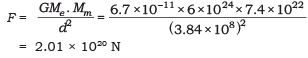Q7. What is effect of shape of earth on value of ‘g’?
Ans.
The Earth is not a perfect sphere. The radius of earth increases from the poles to the equator. Hence, as per relation  g = GM/R2,  where value of ‘g’ is greater at the poles and lesser at the equator.

Q8. An object is just dropped from a certain point to fall freely under gravity. Write the equation of motion.
Ans.
In this case, initial velocity u = 0 and acceleration a = + g. Hence, the equation of motion are:

(i) v = gt            (ii) s = 1/2 gt2           (iii) v2 = 2gs

where ‘s’ is the vertical distance covered below the point from where the object was just dropped.

Q9. What is the distance covered by a freely falling body during the first three seconds of its motion?
Ans.
Here initial velocity u = 0,
acceleration a = g = 9.8 ms–2 and t = 3 s
∴ Vertically downwards distance covered h = 1/2 gt2

=(1/2) × (9.8) × 32 = 44.1 m

Q10. A stone and feather are thrown from a tower. Both the objects should reach the ground at same time but it does not, give reason.
Ans.
As per the motion of object due to ‘g’ gravitational pull of earth, both the bodies are acted upon by the same force of earth but the stone will fall first and then the feather. As feather being lighter, experiences the greater air resistance, so it will reach later.

Q11. Find the weight of Uma, who weighs 36 kg.
Ans.
Mass of Uma m = 36 kg,
∴ Weight of Uma W = mg = 36 × 9.8 N
= 352.8 N

Q12. Why do we feel uneasy when the ferry wheel moves downwards?
Ans.
When we are in the ferry wheel and the swing moves down, our body gets accelerated due to the gravitational forces. This causes weightlessness due to the free fall.

Q13. Derive the formula for the universal law of gravitation.
Ans.
Let two objects A and B of masses M and m, lie at a distance d from each other as shown in the figure.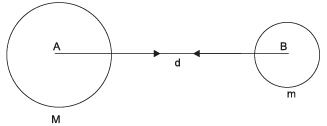Let the force of attraction between two objects be F.
According to the universal law of gravitation, the force between two objects is directly proportional to the product of their masses.
F ∝ Mm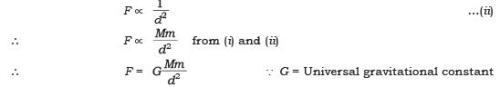Q14. Give the difference between ‘g’ and ‘G’.
Ans.

 ‘g’ ‘G’ 1.It is defined as acceleration due to gravity. 1.It is defined as universal gravitational constant. 2. Value of g = 9.8 2. Value of G = 6.67 × 10−11 3. Unit = m/s2 3. Unit = Nm2/kg2 4. It changes from place to place. At poles value of ‘g’ is more than at equator. 4. It remains constant.

Q15. Find the value of ‘G’, universal gravitational constant.
Ans.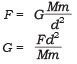For any given value of M and m, mass of two objects, the distance between them is ‘d’ and the force ‘F’ exerted on the two bodies by each other is known as universal gravitational constant. The value of G will always be same. i.e., G = 6.67 × 10−11 Nm2/kg2.
Q16. Find the value of ‘g’, acceleration due to gravity.
Ans.

g= GM/ R2

G = 6.7 × 10−11 Nm2/kg2 (constant)
M = 6 × 1024 kg (Mass of the earth)
R = 6.4 × 10m (Radius of the earth)
On substituting these values in the given equation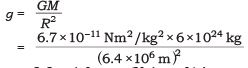= 9.8 m/s2 (Value o f ‘g ’ = acceleration due to gravity)

Q17. Calculate the value of ‘g’ on moon.
Ans.
Moon’s mass = 7.36 × 1022 kg, Radius of moon = 1.74 × 106 m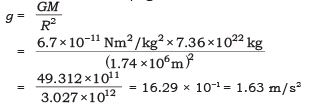∴ The value of ‘g’ on moon = 1.63 m/s2

Q18. A car falls of a ledge and drops to the ground in 0.5 s. Let g = 10 ms–2.
(i) What is its speed on striking the ground?
(ii) What is its average speed during 0.5 s?
(iii) How high is the edge from the ground?
Ans.
Here u = 0, t = 0.5 s  and a = g = + 10 ms–2
(i) ∴ Final speed of car when striking the ground
v = u + at = 0 + gt = 10 × 0.5 = 5 ms–1

(ii) Average speed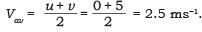(iii) Height of the ledge = total distance covered by car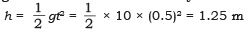Q19. What is centripetal force? Explain it with an example.
Ans.
When a body moves around the other body, the force that causes this acceleration and keeps the body moving along a circular path is acting towards the centre. This force is called the centripetal force. It means centre seeking force.
E.g., tie a stone to a thread and whirl it round as shown in the figure. The stone continues to move round as long as the string is held. On releasing the thread the centre binding force called as centripetal force is no more acting on it, the stone flies off along the straight line. This straight line will be a tangent to the circular path.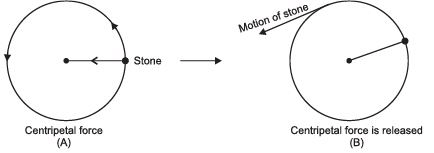Q20. A ship made of iron does not sink but the iron rod sinks in water, why?
Ans.
The iron rod sinks due to high density and less buoyant force exerted by the water on it, but in case of ship the surface area is increased, the upthrust experienced by the body is more. So, it floats on water.

Q21. Camels can walk easily on desert sand but we are not comfortable walking on the sand. State reason.
Ans.
Camel’s feet are broad and the larger area of the feet reduces the force/ pressure exerted by the body on the sand. But when we have to walk on the same sand, we sink because the pressure exerted by our body is not distributed but is directional.

Q22. What is lactometer and hydrometer?
Ans.
Lactometer is a device used to find the purity of a given sample of milk.
Hydrometer is a device used to find the density of liquid.

Q23. The relative density of silver is 10.8. What does this mean?
Ans
. It means that the density of silver is 10.8 times more than that of water.

Q24. The relative density of gold is 19.3. The density of water is 103 kg/m3? What is the density of gold in S.I. unit?
Ans.
Relative density of gold = 19.3

Relative density of gold =  Density of gold/ Density of water

∴ Density of gold = Relative density of gold × Density of water
= 19.3 × 103 kg/m3
= 19300 kg/m3.

Q25. State Archimedes’ principle.
Ans.
Archimedes’ principle—When a body is immersed fully or partially in a fluid, it experiences an upward force that is equal to the weight of the fluid displaced by it. It is used in designing of ships and submarines.

Q26. Two cork pieces of same size and mass are dipped in two beakers containing water and oil. One cork floats on water but another sink in oil. Why?
Ans.
The cork floats on water because the density of cork is less than the density of water, and another cork sinks in the oil because the density of cork is more than the density of oil.

Q27. What are fluids? Why is Archimedes’ principle applicable only for fluids? Give the application of Archimedes’ principle.
Ans.
Fluids are the substances which can flow e.g., gases and liquids are fluids. Archimedes’ principle is based on the upward force exerted by fluids on any object immersed in the fluid. Hence it is applicable only for fluids. Applications of Archimedes’ principle:
(i) It is used in designing of ship and submarine.
(ii) It is used in designing lactometer which is used to determine the purity of milk.
(iii) To make hydrometer that is used to determine the density of liquid.

Q28. Explain why two objects of different mass dropped the same distance from the surface of a planet will experience the same acceleration even though the heavier object experiences more force.
Ans.
The force is greater by the same ratio that the mass is greater. Since F = am, increasing the force and the mass leads to the same acceleration.

The document Short Answers - Gravitation, Science, Class 9 Notes | Study Class 9 Science by VP Classes - Class 9 is a part of the Class 9 Course Class 9 Science by VP Classes.
All you need of Class 9 at this link: Class 9Use Code STAYHOME200 and get INR 200 additional OFF

## Class 9 Science by VP Classes

75 docs|14 tests

Track your progress, build streaks, highlight & save important lessons and more!

,

,

,

,

,

,

,

,

,

,

,

,

,

,

,

,

,

,

,

,

,

,

,

,

,

,